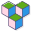CppBuzz# Java program to find number of digits in any number

``````public class Main {

public static void main(String[] args) {
// find number of digits
// 2323 --- number of digit is 4

int num = 123456789;
int count = 0;

while(num != 0) {
num = num/10;
count++;
}
System.out.println("Number of digits : " + count);
}
}``````